# Excel MAXIFS function - get largest value based on multiple criteria

The tutorial shows how to use the MAXIFS function in Excel to get the maximum value with conditions.

Traditionally, when you ever needed to find the highest value with conditions in Excel, you had to build your own MAX IF formula. While not a big deal for experienced users, that might present certain difficulties for novices because, firstly, you should remember the formula's syntax and, secondly, you need to know how to work with array formulas. Luckily, Microsoft has recently introduced a new function that lets us do conditional max an easy way!

## Excel MAXIFS function

The MAXIFS function returns the largest numeric value in the specified range based on one or more criteria.

The syntax of the MAXIFS function is as follows:

MAXIFS(max_range, criteria_range1, criteria1, [criteria_range2, criteria2], …)

Where:

• Max_range (required) - the range of cells where you want to find the maximum value.
• Criteria_range1 (required) - the first range to evaluate with criteria1.
• Criteria1 - the condition to use on the first range. It can be represented by a number, text or expression.
• Criteria_range2 / criteria2, …(optional) - additional ranges and their related criteria. Up to 126 range/criteria pairs are supported.

This MAXIFS function is available in Excel 2019, Excel 2021, and Excel for Microsoft 365 on Windows and Mac.

As an example, let's find the tallest football player in our local school. Assuming the students' heights are in cells D2:D11 (max_range) and sports are in B2:B11 (criteria_range1), use the word "football" as criteria1, and you will get this formula:

`=MAXIFS(D2:D11, B2:B11, "football")`

To make the formula more versatile, you can input the target sport in some cell (say, G1) and include the cell reference in the criteria1 argument:

`=MAXIFS(D2:D11, B2:B11, G1)`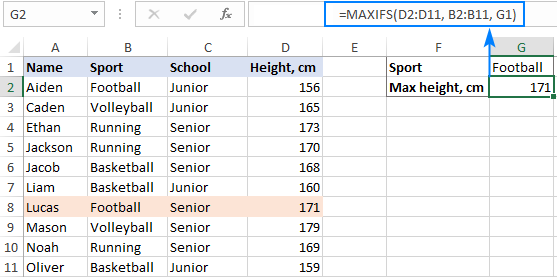Note. The max_range and criteria_range arguments must be of the same size and shape, i.e. contain the equal number of rows and columns, otherwise the #VALUE! error is returned.

## How to use MAXIFS function in Excel - formula examples

As you have just seen, the Excel MAXIFS is quite straightforward and easy to use. However, it does have a few little nuances that make a big difference. In the below examples, we will try to make the most of conditional max in Excel.

### Find max value based on multiple criteria

In the first part of this tutorial, we created a MAXIFS formula in its simplest form to get the max value based on one condition. Now, we are going to take that example further and evaluate two different criteria.

Supposing, you want to find the tallest basketball player in junior school. To have it done, define the following arguments:

• Max_range - a range of cells containing heights - D2:D11.
• Criteria_range1 - a range of cells containing sports - B2:B11.
• Criteria1 - "basketball", which is input in cell G1.
• Criteria_range2 - a range of cells defining the school type - C2:C11.
• Criteria2 - "junior", which is input in cell G2.

Putting the arguments together, we get these formulas:

With "hardcoded" criteria:

`=MAXIFS(D2:D11, B2:B11, "basketball", C2:C11, "junior")`

With criteria in predefined cells:

`=MAXIFS(D2:D11, B2:B11, G1, C2:C11, G2)`

Please notice that the MAXIFS function in Excel is case-insensitive, so you needn't worry about the letter case in your criteria.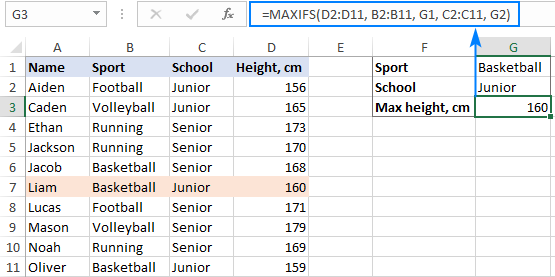In case you plan to use your formula on multiple cells, be sure to lock all the ranges with absolute cell references, like this:

`=MAXIFS(\$D\$2:\$D\$11, \$B\$2:\$B\$11, G1, \$C\$2:\$C\$11, G2)`

This will ensure that the formula copies to other cells correctly - the criteria references change based on the relative position of the cell where the formula is copied while the ranges remain unchanged: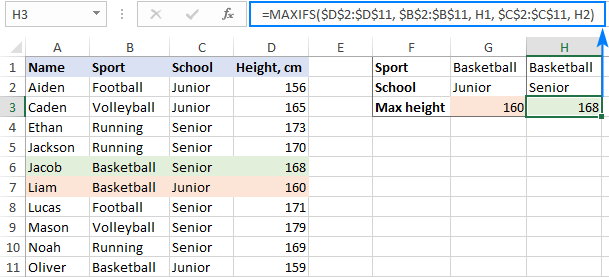As an extra bonus, I will show you a quick way to extract a value from another cell that is associated with the max value. In our case, that will be the name of the tallest person. For this, we will be using the classic INDEX MATCH formula and nest MAXIFS in the first argument of MATCH as the lookup value:

`=INDEX(\$A\$2:\$A\$11, MATCH(MAXIFS(\$D\$2:\$D\$11, \$B\$2:\$B\$11, G1, \$C\$2:\$C\$11, G2), \$D\$2:\$D\$11, 0))`

The formula tells us that the name of the tallest basketball player in junior school is Liam: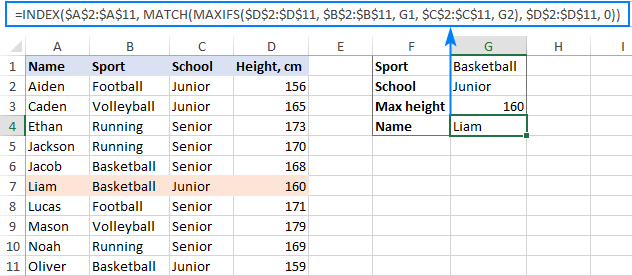### Excel MAXIFS with logical operators

In situation when you need to evaluate numeric criteria, use logical operators such as:

• greater than (>)
• less than (<)
• greater than or equal to (>=)
• less than or equal to (<=)
• not equal to (<>)

The "equal to" operator (=) can be omitted in most cases.

Usually, choosing an operator is not a problem, the trickiest part is to build criteria with the correct syntax. Here's how:

• A logical operator followed by a number or text must be enclosed in double quotes like ">=14" or "<>running".
• In case of a cell reference or another function, use the quotes to begin a string and an ampersand to concatenate the reference and finish the string off, e.g. ">"&B1 or "<"&TODAY().

To see how it works in practice, let's add the Age column (column C) to our sample table and find the maximum height among the boys aged between 13 and 14. This can be done with the following criteria:

Criteria1: ">=13"

Criteria2: "<=14"

Because we compare the numbers in the same column, criteria_range in both cases is the same (C2:C11):

`=MAXIFS(D2:D11, C2:C11, ">=13", C2:C11, "<=14")`

If you do not want to hardcode the criteria in the formula, input them in separate cells (e.g. G1 and H1) and use the following syntax:

`=MAXIFS(D2:D11, C2:C11, ">="&G1, C2:C11, "<="&H1)`

The screenshot below shows the result: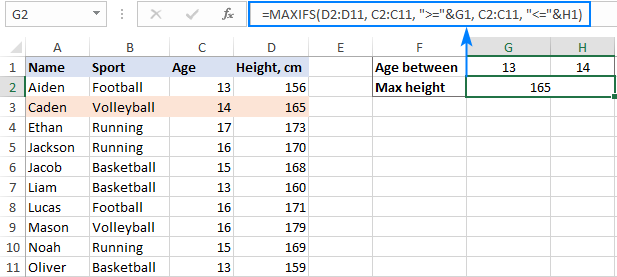Aside from numbers, logical operators can also work with text criteria. In particular, the "not equal to" operator comes in handy when you wish to exclude something from your calculations. For example, to find the tallest student in all sports excluding volleyball, use the following formula:

`=MAXIFS(D2:D11, B2:B11, "<>volleyball")`

Or this one, where G1 is the excluded sport:

`=MAXIFS(D2:D11, B2:B11, "<>"&G1)`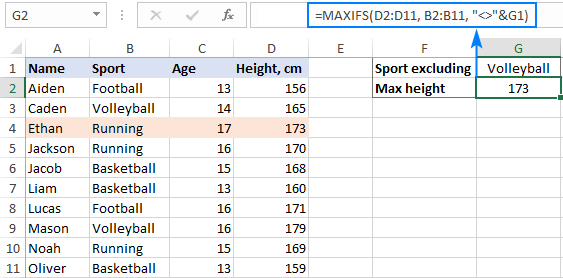### MAXIFS formulas with wildcard characters (partial match)

To evaluate a condition that contains a specific text or character, include one of the following wildcard character in your criteria:

• Question mark (?) to match any single character.
• Asterisk (*) to match any sequence of characters.

For this example, let's find out the tallest guy in game sports. Because the names of all game sports in our dataset end with the word "ball", we include this word in the criteria and use an asterisk to match any previous characters:

`=MAXIFS(D2:D11, B2:B11, "*ball")`

You can also type "ball" in some cell, e.g. G1, and concatenate the wildcard character with the cell reference:

`=MAXIFS(D2:D11, B2:B11, "*"&G1)`

The result will look as follows: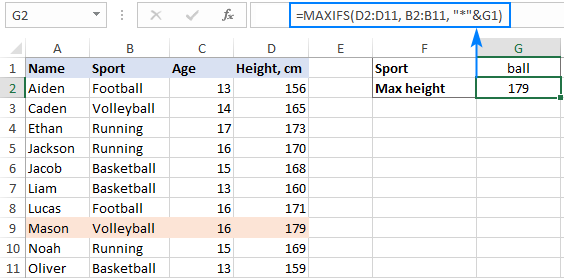### Get max value within a date range

Because dates are stored as serial numbers in the internal Excel system, you work with the dates criteria in the same manner as you work with numbers.

To illustrate this, we will replace the Age column with Date of Birth and try to work out the max height among the boys born in a particular year, say in 2004. To accomplish this task, we need to "filter" the birth dates that are greater than or equal to 1-Jan-2004 and less than or equal to 31-Dec-2004.

When building your criteria, it is important that you provide the dates in the format that Excel can understand:

`=MAXIFS(D2:D11, C2:C11, ">=1-Jan-2004", C2:C11, "<=31-Dec-2004")`

Or

`=MAXIFS(D2:D11, C2:C11, ">=1/1/2004", C2:C11, "<=12/31/2004")`

To prevent misinterpretation, it makes sense to utilize the DATE function:

`=MAXIFS(D2:D11, C2:C11, ">="&DATE(2004,1,1), C2:C11, "<="&DATE(2004,12,31))`

For this example, we will type the target year in G1, and then use the DATE function to supply the dates:

`=MAXIFS(D2:D11, C2:C11, ">="&DATE(G1,1,1), C2:C11, "<="&DATE(G1,12,31))`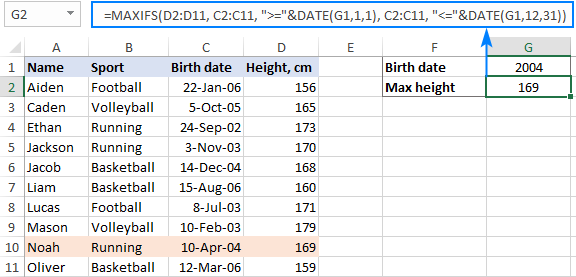Note. Unlike numbers, dates should be enclosed in quotation marks when used in the criteria on their own. For example:

`=MAXIFS(D2:D11, C2:C11, "10/5/2005")`

### Find maximum value based on multiple criteria with OR logic

The Excel MAXIFS function is designed to test the conditions with the AND logic - i.e. it processes only those numbers in max_range for which all the criteria are TRUE. In some situations, however, you may need to evaluate the conditions with the OR logic - i.e. process all the numbers for which any of the specified criteria is TRUE.

To make things easier to understand, please consider the following example. Supposing you want to find the maximin height of the guys who play either basketball or football. How would you do that? Using "basketball" as criteria1 and as "football" criteria2 won't work, because Excel would assume that both criteria should evaluate to TRUE.

The solution is to make 2 separate MAXIFS formulas, one per each sport, and then use the good old MAX function to return a higher number:

`=MAX(MAXIFS(C2:C11, B2:B11, "basketball"), MAXIFS(C2:C11, B2:B11, "football"))`

The screenshot below shows this formula but with the criteria in predefined input cells, F1 and H1: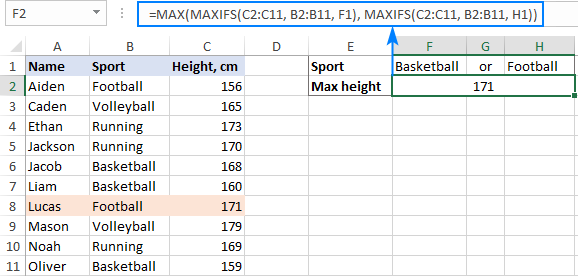Another way is to use a MAX IF formula with OR logic.

## 7 things to remember about Excel MAXIFS

Below you will find a few remarks that will help to improve your formulas and avoid common errors. Some of these observations have already been discussed as tips and notes in our examples, but it might be helpful to get a short summary of what you've already learned:

1. The MAXIFS function in Excel can get the highest value based on one or multiple criteria.
2. By default, Excel MAXIFS works with the AND logic, i.e. returns the maximum number that meets all of the specified conditions.
3. For the function to work, the max range and criteria ranges must have the same size and shape.
4. The SUMIF function is case-insensitive, i.e. it does not recognize the letter case in text criteria.
5. When writing a MAXIFS formula for multiple cells, remember to lock the ranges with absolute cell references for the formula to copy correctly.
6. Mind the syntax of your criteria! Here are the main rules:
• When used on their own, text and dates should be enclosed in quotation marks, numbers and cell references should not.
• When a number, date or text is used with a logical operator, the whole expression must be enclosed in double quotes like ">=10"; cell references and other functions must be concatenated by using an ampersand like ">"&G1.
7. MAXIFS is only available in Excel 2019 and Excel for Office 365. In earlier versions, this function is not be available.

That's how you can find the maximum value in Excel with conditions. I thank you for reading and hope to see you on our blog soon!

Excel MAXIFS formula examples (.xlsx file)

## You may also be interested in

1. I'm trying to use the formula maxifs(Sheet2!\$A\$2:A\$21-A2, Sheet2!\$A\$2:A\$21-A2, "<=0") but it doesn't work. Excel says there is a problem with this formula.

• Hi! The problem is Sheet2!\$A\$2:A\$21-A2. This is an invalid expression. I can't advise you as I don't know what you wanted to do. The MAXIFS function argument is a range of values, but not a formula. Please read the above article carefully.

• Thanks for your reply. I'm trying the find the closest date to a specific date in cell A2 from the range Sheet2!A2:A21.

• Hi! To get the closest date to a specific date in cell A2, use MIN function:

=INDEX(A3:A21, MATCH(MIN(ABS(A3:A21-A2)), ABS(A3:A21-A2),0))

2. I need a formula,To filter data for a list of students from different sections to find the highest score per section and give the name of the student with the grade and id.

I create this formula :

=VLOOKUP(MAX(FILTER(FILTER(A2:J221,(C2:C221=S6)*(A2:A221=S7),0),{0,0,0,0,1,0,0,0,0,0})),E2:J221,{1,3,5,4,6},0)

But the problem is that its not giving me the highest grade persection , its giving me the highest grade in all the sections.

• Hi!
To find the maximum score for a condition, use the MAXIFS function instead of MAX. Read the recommendations above carefully.

3. If I need to find the highest grade per grade and per subject, what formula I can use ?
Thank you.

• Hi!

• If I have a list of students from different sections I need to find the highest score per section for two different subjects and give the name of the student with the grade. What formula? Can you help me pls.

Thank you

• Hi!
To find the highest score, use recommendations from this article above. To find a name of the student with this highest score, use INDEX MATCH function. I don't have your data so I can't give you the formula. Follow the guidelines in these articles.

4. Hi! I'm trying to get the max Week value of one (1), based on the max values of both Year and Month (2029 and 1), for the three records below. The formula works as expected when there is only one range/criteria combination but returns the value zero (0), which means a record can't be found, as shown in the formula below.

Could you please provide a corrected MAXIFS and an explanation as to why my formula isn't working as expected.

Year,Month,Week
2028,1,2
2028,12,52
2029,1,1

=MAXIFS ([Week], [Year], MAX([Year]), [Month], MAX([Month]))

• Hello!
The MAXIFS formula returns 0 because the maximum year (2029) and maximum month (12) are on different rows.
Use instruction - MAX IF formula with multiple criteria.
Try this formula -

=MAX(IF((Table1[Year]=MAX(Table1[Year]))*((MAX(Table1[Year])=Table1[Year])*Table1[Month]),Table1[Week]))

• Thanks for your prompt response and accurate formula! I thought that that was what was going on with my formula code.

If Microsoft doesn't have a function to does what I wanted to do, maybe they will create a DMaxIfs function that does that. Hopefully they are reading this.

Ultimately in the end, I needed something usable as a VBA code snippet. This morning I had a rethink and all I really needed was the Year and Week fields to get the max value for Week.

If I hadn't figured it out after frying too many brain cells, I would have definitely used your formula code and then wrote VBA code to that cell address. Here's what I came up with for Excel and VBA. Hopefully this helps someone down the road.

Worksheet formula...
=MAXIFS(TableRangeName[WeekRangeName],TableRangeName[YearRangeName],MAX(TableRangeName[YearRangeName]))

VBA code snippet...

Dim FY, RW As Integer
' Get Max values for records using named ranges
' TableRangeName not needed since named ranges are unique to the workbook
FY = WorksheetFunction.Max([YearRangeName])
RW = WorksheetFunction.MaxIfs([WeekRangeName], [YearRangeName], WorksheetFunction.Max([YearRangeName))
End Sub

5. What would the MAXIFS formula for the following example below look like?

1 Absent ("0" or "1" is displayed using [=IF((AND('Severity Rating'.I3="No",'Severity Rating'.I4="No",'Severity Rating'.I15="No")),"1","0")])
2 Mild ("2" is displayed using [=IF('Severity Rating'.I3="Yes";"2";"0")])
3 Moderate ("3" is displayed using [=IF('Severity Rating'.I4="Yes";"3";"0")])
4 Severe ("4" is displayed using [=IF('Severity Rating'.I5="Yes";"4";"0")])

I want to create a function to identify the max number (4 in this case) and sum all the severity ratings in each category (the above is just one category).

Thanks!

• Hello!
Your formulas return text, not numbers. Instead of "1" use 1 and so on.
To find the sum of the ratings in a given category, use the SUMIF function instruction.

• That worked!! Thank you!

6. If i want the value to be returned for multiple sheets how this formula can be changed?

• Hi!
What formula are you talking about and what data do you want to return?

7. Is it possible to find MAX difference (in numbers) between 2 columns i.e. In Jan 2022 Mark's number was 170 and Philip's number was 172. Now in Feb 2022 Mark's number is 200 and Philip's number is 220. I want a formula to find the max difference between Jan & Feb numbers like here Philip's numbers increased by 48 numbers (highest between 2) and it should return Philip's name.

• Hi!
You have not specified how your data is written. For example, the formula might look like this:

=IF(A3-A2 > B3-B2,A1,B1)

If this is not what you wanted, please describe the problem in more detail.

8. Index / Maxifs will return the first record found with matching value ... If Ethan height was 171.. Maxifs formula becomes irrelevant as Index will return Ethan's name

isn't it ?

9. Trying to figure out how to write a MAX IF condition to cover all scenarios.
If a calculated value is a negative number, then default to '0'. If calculated value is a positive number, then display a whole number that doesn't round up ('20.8' should display as '20'). The formulas below work independently, but haven't been able to blend the two into a single formula.

=MAX(0,220-D12)*E12

=INT((220-D12)*E12)

• Hello!
If I understand your task correctly, the following formula should work for you:

=IF(A2>0,ROUNDDOWN(A2,0),0)